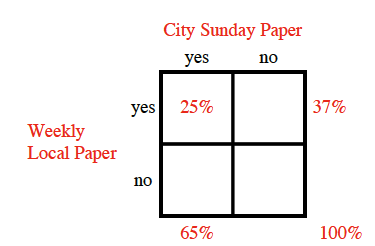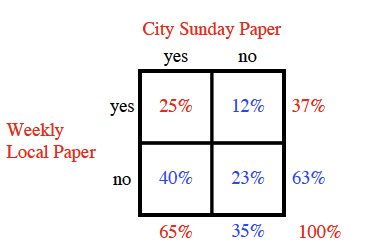### Home > CCA > Chapter 10 > Lesson 10.1.1 > Problem10-12

10-12.

In a certain small town, 65% of the residents subscribe to the city Sunday paper, 37% subscribe to the weekly local paper, and 25% subscribe to both papers.

1. What are the two variables? Create a two-way table.

A generic rectangle where the outside upper left edge is 'yes' and the lower left edge is 'no'. The outer top left edge is 'yes' and the top right is 'no'. The bottom left edge is 65 percent. The right outer upper edge is 37 percent. The right bottom outer corner is 100 percent. The interior is labeled as follows: Upper left box is 25 percent. The top title is 'City Sunday Paper'. The left side title is 'Weekly Local Paper'.2. If a resident is selected at random, what is the probability he or she subscribes to at least one of the two papers?

Which three boxes in the two-way table represent someone subscribing to at least one paper? The same generic rectangle with the following new components entered in a different color from the rest. The bottom right edge is 35 percent. The right outer lower edge is 63 percent. For the interior, the upper right is 12 percent, the lower left is 40 percent and lower right is 23 percent.

$25\%+12\%+40\%=77\%$

1. Charlie’s neighbor subscribes to a paper. What is the probability that he takes the Sunday paper?

• This is the probability of someone subscribing to the Sunday paper given the information that they subscribe to at least one paper. Use your answer from part (b).

$\frac{65}{77}=?$